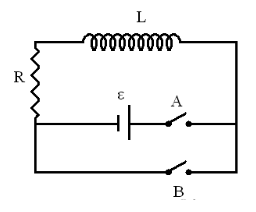# Problem: An RL circuit is shown on the right. L = 2.2 J, R = 258 Ω, ε = 4.5 VPart (a) Switch A is closed at t = 0. Express the current I in the circuit as a function of time in terms of L, R, and ε.Part (b) Whats is the direction of the current I, clockwise or counterclockwise?

###### FREE Expert Solution
94% (285 ratings)View Complete Written Solution
###### Problem Details

An RL circuit is shown on the right. L = 2.2 J, R = 258 Ω, ε = 4.5 VPart (a) Switch A is closed at t = 0. Express the current I in the circuit as a function of time in terms of L, R, and ε.

Part (b) Whats is the direction of the current I, clockwise or counterclockwise?